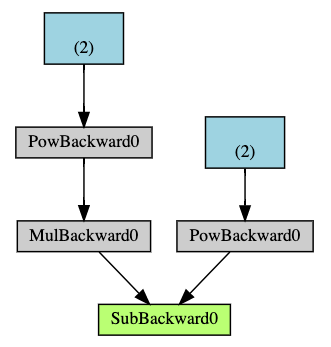# [源码解析]深度学习利器之自动微分(3) --- 示例解读

## 0x01 概述

### 1.2 如何应用

• 运行请求的操作以计算结果张量。

• 利用.grad_fn计算每个张量的梯度，并且依据此构建出包含梯度计算方法的反向传播计算图。
• 将梯度累积在各自的张量.grad属性中，并且使用链式法则，一直传播到叶张量。
• 每次迭代都会重新创建计算图，这使得我们可以使用Python代码在每次迭代中更改计算图的形状和大小。

## 0x02 示例

### 2.2 实例解读 1

#### 2.2.1 代码

import torch

O = 3*a**3
P = b**2
Q = O - P

Q = 3*a**3 - b**2



tensor(36.)
tensor(-12.)
tensor(36.)
tensor(-12.)

((<MulBackward0 object at 0x000001374DE6C308>, 0), (<PowBackward0 object at 0x000001374DE6C288>, 0))
((<PowBackward0 object at 0x000001374DE6C288>, 0), (None, 0))
None
None


$Q = 3a^3 - b^2$

$\frac{∂Q}{∂a} = 9a^2 \\\frac{∂Q}{∂b} = -2b$

#### 2.2.2 分析

• a 是 2，b 是 6， Q 是 tensor(-12., grad_fn=<SubBackward0>)

• Q对于 a 的积分是：$$\frac{∂Q}{∂a} = 9a^2$$ = 36。
• Q对于b的积分是 $$\frac{∂Q}{∂b} = -2b$$ = -12。

$\frac{∂Q}{∂Q} = 1$

external_grad = torch.tensor([1., 1.])### 2.3 实例解读 2

#### 2.3.1 示例代码

import torch

x = torch.ones(5)  # input tensor
y = torch.zeros(3)  # expected output
z = torch.matmul(x, w)+b
loss = torch.nn.functional.binary_cross_entropy_with_logits(z, y)


#### 2.3.2 张量、函数和计算图print('Gradient function for z =', z.grad_fn)


Gradient function for z = <AddBackward0 object at 0x7f4dbd4d3080>
Gradient function for loss = <BinaryCrossEntropyWithLogitsBackward object at 0x7f4dbd4d3080>


#### 2.3.3 计算梯度

loss.backward()


tensor([[0.1881, 0.1876, 0.0229],
[0.1881, 0.1876, 0.0229],
[0.1881, 0.1876, 0.0229],
[0.1881, 0.1876, 0.0229],
[0.1881, 0.1876, 0.0229]])
tensor([0.1881, 0.1876, 0.0229])


• 我们只能获取在计算图叶子节点的requires_grad属性设置为True时候得到该节点的grad属性。我们没法得到们图中的所有其他节点的梯度。
• 出于性能原因，我们只能在给定的计算图之上使用backward执行一次梯度计算 。如果我们需要在同一个图上多次调用backward，则需要在backward调用时候设置 retain_graph=True

#### 2.3.4 禁用梯度跟踪

z = torch.matmul(x, w)+b

z = torch.matmul(x, w)+b


True
False


z = torch.matmul(x, w)+b
z_det = z.detach()


False


• 将神经网络中的某些参数标记为冻结参数。这是微调预训练网络的一个非常常见的场景。
• 在仅进行前向传递时加快计算速度，因为对不跟踪梯度的张量进行计算会更有效。

## 0x03 逻辑关系

• 图表示计算任务。PyTorch把计算都当作是一种有向无环图，或者说是计算图，但这是一种虚拟的图，代码中没有真实的数据结构
• 计算图由节点（Node）边（Edge）组成。
• 节点（Node）代表了运算操作。
• 一个节点通过边来获得 0 个或多个 Tensor，节点执行计算之后会产生 0 个或多个 Tensor
• 节点的成员变量 next_functions 是一个 tuple 列表，此列表就代表本节点要输出到哪些其他 Function。列表个数就是这个 grad_fn 的 Edge 数目，列表之中每一个 tuple 对应一条 Edge 信息，内容就是 (Edge.function, Edge.input_nr)。
• 边（Edge）就是运算操作之间的流向关系。
• Edge.function ：表示此 Edge 需要输出到哪一个其他 Function。
• Edge.input_nr ：指定本 Edge 是 Function 的第几个输入。
• 使用张量（ Tensor） 表示数据，就是在节点间流动的数据，如果没有数据，计算图就没有任何意义。

+---------------------+              +----------------------+
| SubBackward0        |              | PowBackward0         |
|                     |      Edge    |                      |  Edge
|   next_functions  +-----+--------> |     next_functions +----------> ...
|                     |   |          |                      |
+---------------------+   |          +----------------------+
|
|
|          +----------------------+
|  Edge    | MulBackward0         |
+--------> |                      |  Edge
|     next_functions +----------> ...
|                      |
+----------------------+


## 0xFF 参考

pytorch学习笔记（十三）：backward过程的底层实现解析

PyTorch的初始化

pytorch的自动求导机制 - 计算图的建立

PyTorch的优化器

PyTorch的分布式

PyTorch的Tensor（下）

PyTorch的Tensor(中)

PyTorch的Tensor（上）

PyTorch的动态图(下)

PyTorch的动态图(上)